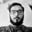Related Tags

python
community creator
mean
median
mode

# Basic statistics using PythonAman Anand

“Statistics is like a high-caliber weapon: helpful when used correctly and potentially disastrous in the wrong hands.”

Statistics can be used to explain many things like DNA testing, factors associated with diseases (like cancer or heart disease), or the idiocy of playing the lottery. Statistics are present everywhere in our day-to-day life, from batting averages in cricket to US presidential election polls, from weather prediction probabilities to data science and machine learning. Statistics is the branch of mathematics that deals with the collection, organization, analysis, interpretation, and representation of data.

Machine Learning which is the most sought-after tech in the present time, and is basically the analysis of statistics to help computers make decisions based on repeatable characteristics found in the data.In this post, we will be seeing the basics of statistics like mean, median, mode, and standard deviation being used with the help of Python.

## Mean

Here, mean refers to the average of numbers, which means that we add the numbers and divide them by the total number of items present. The code for this is:

a=[11, 21, 34, 22, 27, 11, 23, 21]

mean = sum(a)/len(a)
print (mean)

We can also calculate the mean using numpy. The code is:

import numpy as np
a =[11, 21, 34, 22, 27, 11, 23, 21]
mean = np.mean(a)
print (mean)

## Median

Median is the middle term that occurs in a sorted array. For an odd number of elements, the median is the middle term, and for an even number of elements, the median is the average of two terms in the middle.

def median(nums):
nums.sort()
if len(nums)%2 == 0:

return int(nums[len(nums)//2-1]+nums[len(nums)//2])/2
else:
return nums[len(nums)//2]

a =[11, 21, 34, 22, 27, 11, 23, 21]
print (median(a))

The numpy code for finding median is:

import numpy as np

a =[11, 21, 34, 22, 27, 11, 23, 21]
print(np.median(a))

## Mode

Mode refers to the element that has the highest frequency in a list of elements. It is the element that occurs the maximum number of times. The Python implementation to find mode is given below.

from collections import Counter

a =[11, 21, 34, 22, 27, 11, 23, 21]
data = dict(Counter(a))
mode = [k for k, v in data.items() if v == max(list(data.values()))]
print (mode)

Scipy provides a method to find the mode of an array or list of elements. One drawback of this method is that it only gives one solution even if the data is multimodal.

from scipy import stats

a =[11, 21, 34, 22, 27, 11, 23, 21]
print (stats.mode(a))

## Quartiles

The quartiles divide data into four parts. The first part comprises of start to first quartile(Q1), the second part comprises of the first quartile to second quartile(Q2), the third part is Q2 to Q3, and the fourth part is Q3 to end. The data must be sorted in order to find the quartiles. The code for finding the quartiles is given below (the median function is the function used above in the median section):

def quartiles(nums):
nums=sorted(nums)
Q1 = median(nums[:len(nums)//2])
Q2 = median(nums)
if len(nums)%2 == 0:
Q3 = median(nums[len(nums)//2:])
else:
Q3 = median(nums[len(nums)//2+1:])
return Q1,Q2,Q3

def median(nums):
nums.sort()
if len(nums)%2 == 0:

return int(nums[len(nums)//2-1]+nums[len(nums)//2])/2
else:
return nums[len(nums)//2]

a =[11, 21, 34, 22, 27, 11, 23, 21]
print (quartiles(a))

## Standard deviation

Standard deviation is the measure of the dispersion or spread of data. It is the square root of Variance. The simple Python implementation to find standard deviation is given below:

a =[11, 21, 34, 22, 27, 11, 23, 21]
n=len(a)
std=(sum(map(lambda x: (x-sum(a)/n)**2,a))/n )**0.5
print(std)

The numpy function to find the standard deviation is:

import numpy as np

a =[11, 21, 34, 22, 27, 11, 23, 21]
print (np.std(a))

RELATED TAGS

python
community creator
mean
median
mode

CONTRIBUTORAman Anand